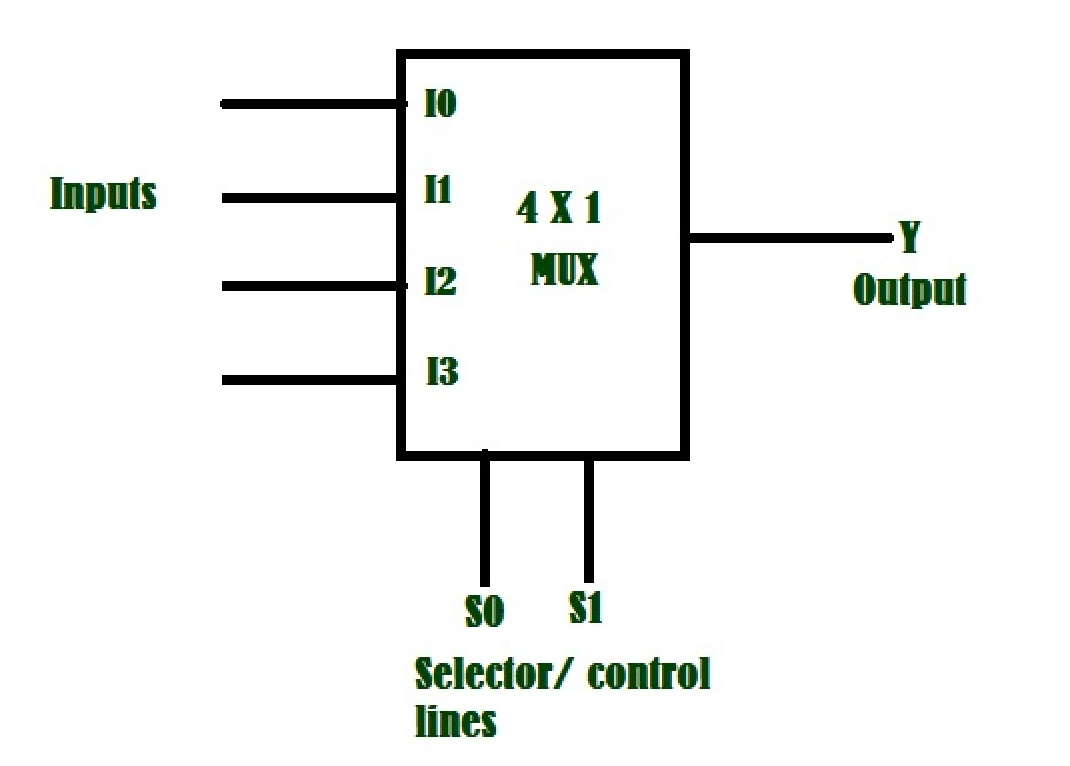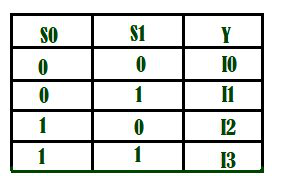Open in App
Not now

# Python program to implement 2:4 Multiplexer

• Difficulty Level : Expert
• Last Updated : 11 Aug, 2021

Prerequisite :  Multiplexers in Digital Logic

Introduction :
It is a combinational circuit which have many data inputs and single output depending on control or select inputs.​ For N input lines, log n (base2) selection lines, or we can say that for 2n input lines, n selection lines are required. Multiplexers are also known as “Data n selector, parallel to serial convertor, many to one circuit, universal logic circuit​”. Multiplexers are mainly used to increase amount of the data that can be sent over the network within certain amount of time and bandwidth.Here if we have four inputs, as mentioned above we will have log 4(base 2)=2 input lines . so for every unique value of the multiplexer selection S0 and S1 we will select a input line and give it as output. The truth table given below explains the selection of the input port.Generally the decimal value of the S0S1 gives the selection port . we are given with s[ ] (selectors) and I[ ](inputs) our task is to select the input port based on selectors and print the value in that port as output.
For binary to decimal conversion refer – Binary to decimal conversion

Example –

Input : I[ ]={0,1,1,1]
s[ ]={1,0}

Output : 1

Explanation : Here s=1,s=0, so its decimal value will be (1*(2**1))+(0*(2**0))=2. so we have to output the value given at pin I2. That is I=1 so the output is 1.

Input : I[ ]={1,1,1,0}
s[ ]={1,1}

Output : 0

Explanation : Here s=1,s=1, so its decimal value will be (1*(2**1))+(1*(2**0))=3. so we have to output the value given at input I3. That is I=0 so the output is 0.

Input : I[ ]={0,1,1,0}
s[ ]={0,1}

Output : 1

Explanation : Here s=0,s=1, so its decimal value will be (0*(2**1))+(1*(2**0))=1. so we have to output the value given at input I1. That is I=1 so the output is 1.

Approach :

• Here we have to convert selectors to decimal value.
• so to convert selector lines to decimal number ,we should multiply (s*(2**1))+(s*(2**0)).
• Getting that  index of decimal value from the I[ ] input array  gives the output.

## Python

 `# python program to implement multiplexer`` ``# Function to print output``def` `Multiplexer(I,s):``    ``#decimal value of s``    ``d``=` `(s[``0``] ``*` `2``) ``+` `(s[``1``] ``*` `1``)``    ``# getting the output of decimal value from inputs ``    ``b ``=` `I[d];``    ``#print the output``    ``print``(b)``# Driver code``I``=``[``1``,``0``,``1``,``0``]``s``=``[``1``,``0``]``#passing I and s to function``Multiplexer(I,s)`

My Personal Notes arrow_drop_up